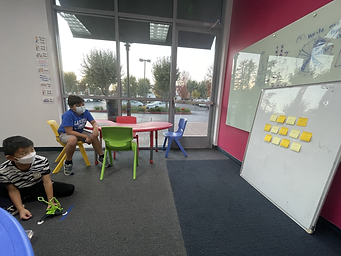Ms. Caitlyn

Target 1​

Lesson Type:

Continuation

Data Probability

:

Interpreting Data

Construct and read graphs from given information to solve problems.

1:

Understand that the data in a graph can also be put into a data table.

5th

Vocabulary:

Trials, Data Table

Activities:

• Students catapulted balls onto a score board and kept score for twelve trials.
• Students inputted their scores into a data table.Home Exploration

Guiding Questions:Absent Students:

Daxton, Akaash

Target 2

:

1:

Understand that different strategies are used for finding the median of an even and an odd set of items.

2:

Understand that median is the middle number in a list of numbers (that are listed in order from least to greatest).

3:

Understand that the mean is the sum of the items divided by the number of items.

4:

Understand that the mode is the number(s) that occur most often.

5:

Find the mean, median, and mode for the same data set.

6th

Vocabulary:

Mean, Median, Mode, Measures of Central Tendency, Data Set

Activities:

• Students found the mean, median, and mode of their scores.
• Students drew cards and found the mean, median, and mode of the numbers on the cards.Home Exploration

Guiding Questions:Target 3

:

Vocabulary:

Activities:Home Exploration